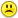+0

# Water is leaking out of an inverted conical tank at a rate of 11,000 cm3/min at the same time that water is being pumped into the tank at a

0
3
5869
13

Water is leaking out of an inverted conical tank at a rate of 11,000 cm3/min at the same time that water is being pumped into the tank at a constant rate. The tank has height 6 m and the diameter at the top is 4 m. If the water level is rising at a rate of 20 cm/min when the height of the water is 2 m, find the rate at which water is being pumped into the tank. (Round your answer to the nearest integer.)

Oct 24, 2014

#1
+10

The radius of the water surface is 1/3 the height of the water.

let the height be h and the radius be h/3     (in cm)

$$\\V=\frac{1}{3}\pi r^2 h\\\\ V=\frac{1}{3}\pi(\frac{h}{3})^2 h\\\\ V=\frac{\pi h^3}{27} \\\\ \frac{dV}{dh}=\frac{\pi h^2}{9}$$

$$\\When \;\;h=200\qquad radius=200/3\\\\ V=\frac{\pi\times 200^3}{27}=\frac{8\times 10^6 \pi}{27}\\\\ \frac{dV}{dh}=\frac{\pi h^2}{9}=\frac{\pi\times 200^2}{9}=\frac{4\times 10^4 \pi}{9}\\\\ \frac{dh}{dt}=20\\\\\\$$

$$\\\frac{dV}{dt}=\frac{dV}{dh}\times \frac{dh}{dt}\\\\ \frac{dV}{dt}=\frac{4\times 10^4 \pi}{9}\times 20\\\\ \frac{dV}{dt}=\frac{80\times 10^4 \pi}{9}\\\\\\ \frac{80\times 10^4 \pi}{9}=\frac{dV_{in}}{dt}-\frac{dV_{out}}{dt}\\\\ \frac{80\times 10^4 \pi}{9}=\frac{dV_{in}}{dt}-11000\\\\ \frac{dV_{in}}{dt}=\frac{80\times 10^4 \pi}{9}+11000\\\\ \frac{dV_{in}}{dt}=290,253\;\;cm^3/min\\\\$$

I think the water is being pumped into the tank at the rate of  290 litres per minute to the nearest litre. (edited)

---------------------------------------------------------

LancelotLink will probably have some great retort for my benefit  LOL

----------------------------------------------------------

Okay LancelotLink has has some fun at my expense.  He got Chimp Ayumu to do it.  She is one smart Chimp.

Our answers are still different.  Mine does look rather huge.I'll have to look some more!

How annoying.----------------------------------------------------------

It's all fixed now and I have a very good excuse!

Someone changed the metric system.  Who did that!

Now that there are only 100cm in a metre instead of my original 1000cm in a metre, it works perfectly.

DOH!Oct 25, 2014

#1
+10

The radius of the water surface is 1/3 the height of the water.

let the height be h and the radius be h/3     (in cm)

$$\\V=\frac{1}{3}\pi r^2 h\\\\ V=\frac{1}{3}\pi(\frac{h}{3})^2 h\\\\ V=\frac{\pi h^3}{27} \\\\ \frac{dV}{dh}=\frac{\pi h^2}{9}$$

$$\\When \;\;h=200\qquad radius=200/3\\\\ V=\frac{\pi\times 200^3}{27}=\frac{8\times 10^6 \pi}{27}\\\\ \frac{dV}{dh}=\frac{\pi h^2}{9}=\frac{\pi\times 200^2}{9}=\frac{4\times 10^4 \pi}{9}\\\\ \frac{dh}{dt}=20\\\\\\$$

$$\\\frac{dV}{dt}=\frac{dV}{dh}\times \frac{dh}{dt}\\\\ \frac{dV}{dt}=\frac{4\times 10^4 \pi}{9}\times 20\\\\ \frac{dV}{dt}=\frac{80\times 10^4 \pi}{9}\\\\\\ \frac{80\times 10^4 \pi}{9}=\frac{dV_{in}}{dt}-\frac{dV_{out}}{dt}\\\\ \frac{80\times 10^4 \pi}{9}=\frac{dV_{in}}{dt}-11000\\\\ \frac{dV_{in}}{dt}=\frac{80\times 10^4 \pi}{9}+11000\\\\ \frac{dV_{in}}{dt}=290,253\;\;cm^3/min\\\\$$

I think the water is being pumped into the tank at the rate of  290 litres per minute to the nearest litre. (edited)

---------------------------------------------------------

LancelotLink will probably have some great retort for my benefit  LOL

----------------------------------------------------------

Okay LancelotLink has has some fun at my expense.  He got Chimp Ayumu to do it.  She is one smart Chimp.

Our answers are still different.  Mine does look rather huge.I'll have to look some more!

How annoying.----------------------------------------------------------

It's all fixed now and I have a very good excuse!

Someone changed the metric system.  Who did that!

Now that there are only 100cm in a metre instead of my original 1000cm in a metre, it works perfectly.

DOH!Melody Oct 25, 2014
#2
+5

Chimp Ayumu thinks you climbed up the wrong tree.:)

She says it is 290,253 cm3/min

Chimp Loki is not here to do the LaTex.

It’s the weekend, so I only care if there is enough water for swimming!We Chimps know what’s really important in life.

Oct 25, 2014
#3
+5

Well Lancelotlink you have a whole team working with you.

Is that akin to cheating?

Maybe that is why chimp starts with ch ??Oct 25, 2014
#4
+5

Yes! That's true. Any member on the board can match wits with an average human, but our collective numbers are the only way we can compete with high-level humans.

You might say we are a sodality of a sedulity. :)

Oct 25, 2014
#5
0

Yes, well, you are smarter than Yogi.  That's for sure.  :)

(and he is smarter than the average bear)

Oct 25, 2014
#6
+5

Those chimps aren't monkeying around.......and they'd better not be.....

By my calculations, that tank holds pi/3*(200cm)2*(600cm) ≈ 25,132,741 cm3 of water

Which means that, if Melody's calculations are accurate......that the whole tank will be filled in about  (25,132,741 / 27, 936, 268) min ≈ 54 seconds

Thus, taking into account the "Primate Float Factor" and "Imperial Minims," means that the chimps will be "washed" out of the tank after a "Total Recreation Time" of less than one minute.....!!!!!

Someone is NOT going to be a happy camper........Oct 25, 2014
#7
+5

Here's my contribution:.

Oct 25, 2014
#8
+5

A somewhat simpler way of tackling at this problem is as follows:.

Oct 25, 2014
#9
+5

This time I will have 100cm in a metre instead of the 1000cm in a metre that I had before.

That might help  :))

Oct 25, 2014
#10
0

I understood your method, Alan......but, am I misreading this?? You say "r" at the "time of interest" = 1m ???  I thought it would be 2/3m based on similar triangles.....Oct 25, 2014
#11
+5

Good job, Alan and Melody !!!Oct 25, 2014
#12
+5

Thanks ChrisOct 25, 2014
#13
+5

Chris wrote: "I understood your method, Alan......but, am I misreading this?? You say "r" at the "time of interest" = 1m "

Well spotted Chris - you are right:  r = 2/3 m.  The r = 1m is there because I inadvertently set the whole worksheet to round all numbers to the nearest integer, instead of just setting the final result to be rounded to the nearest integer.

The correct value is used in the calculation though.

.

Oct 25, 2014